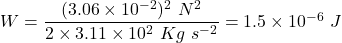## A cylindrically shaped piece of collagen (a substance found in the body in connective tissue) is being stretched by a force that increases f

Question

A cylindrically shaped piece of collagen (a substance found in the body in connective tissue) is being stretched by a force that increases from 0 to 3.06 10-2 N. The length and radius of the collagen are, respectively, 2.7 and 0.093 cm, and Young’s modulus is 3.10 106 N/m2.
(a) If the stretching obeys Hooke’s law, what is the spring constant k for collagen?
(b) How much work is done by the variable force that stretches the collagen?

in progress 0
6 months 2021-08-31T05:17:39+00:00 1 Answers 0 views 0

Part(a): The value of the spring constant is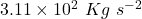.

Part(b): The work done by the variable force that stretches the collagen is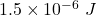.

Explanation:

Part(a):

If ‘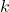‘ be the force constant and if due the application of a force ‘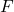‘ on the collagen ‘‘ be it’s increase in length, then from Hook’s law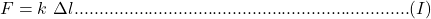Also, Young’s modulus of a material is given by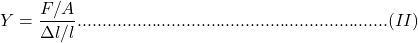where ‘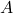‘ is the area of the material and ‘‘ is the length.

Comparing equation (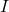) and (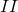) we can write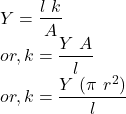Here, we have to consider only the circular surface of the collagen as force is applied only perpendicular to this surface.

Substituting the given values in equation (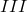), we have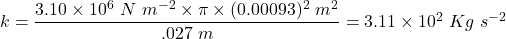Part(b):

We know the amount of work done (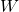) on the collagen is stored as a potential energy (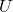) within it. Now, the amount of work done by the variable force that stretches the collagen can be written as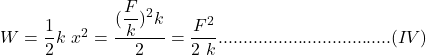Substituting all the values, we can write